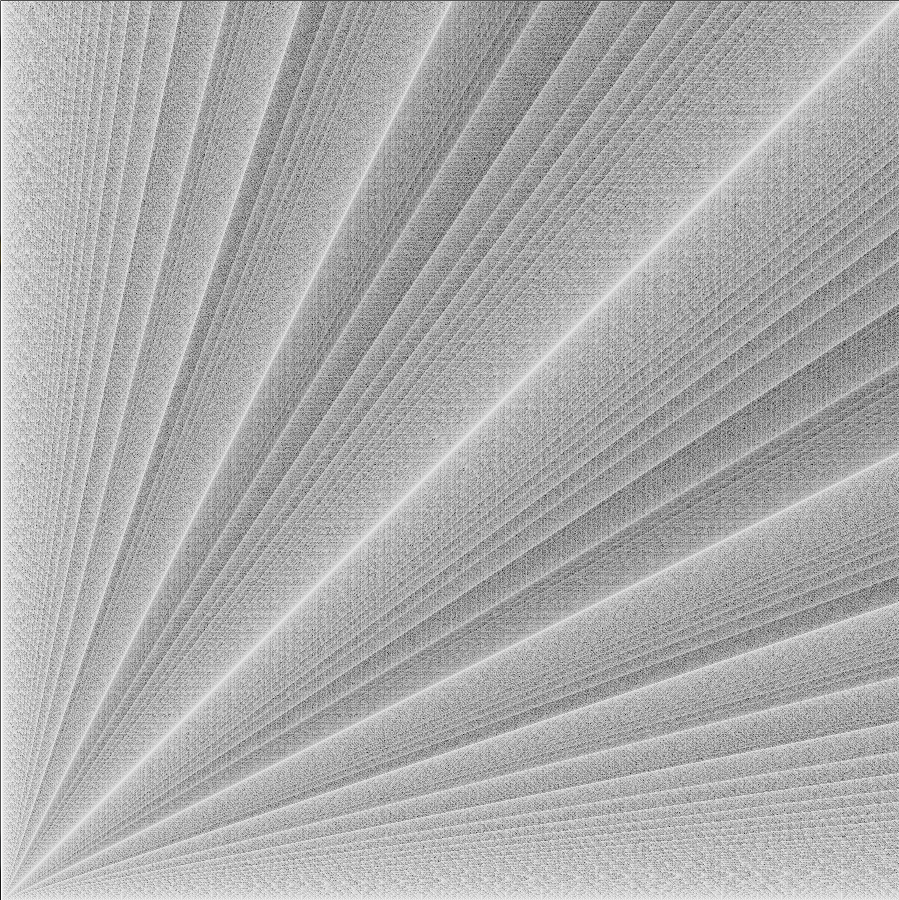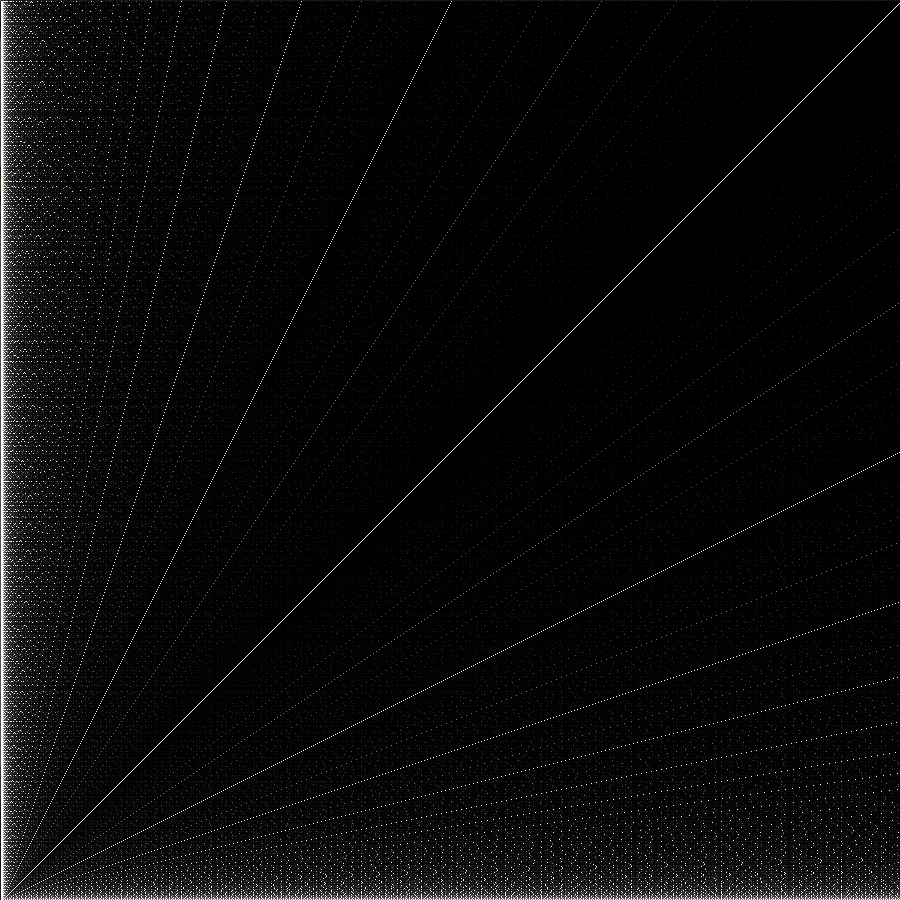# Euclid GCD

I saw this toot by Matt yesterday morning and I loved the visual of the color based on the number of steps of Euclid’s GCD algorithm. The algorithm is pretty straightforward and it’s a nice example for either using recursion or using a loop. The coloring is fun to mess with too. The coding went quickly for me because I already had the code for breaking a 1D pixel array (why processing??) into x and y coordinates.
There’s two variations:
Here’s the link for the GCD steps version.And here’s the link for the GCD version where the closer the GCD is to the minimum of x and y, the more white the pixel is.Here’s the recursive algorithm:

```int euclidGCD(int x, int y)
{
if (y == 0)
{
return x;
}
else if (x >= y && y > 0)
{
return euclidGCD(y,(x%y));
}
else
{
return euclidGCD(y,x);
}
}
```

Here’s the loop algorithm to count the number of steps:

```int euclidGCDsteps(int x, int y)
{
int t;
int steps = 0;
if (x >= y)
{
while (y != 0)
{
t = y;
y = x % y;
x += t;
steps++;
}
}
else
{
return euclidGCDsteps(y,x);
}
return steps;
}
```
This entry was posted in Full Posts. Bookmark the permalink.